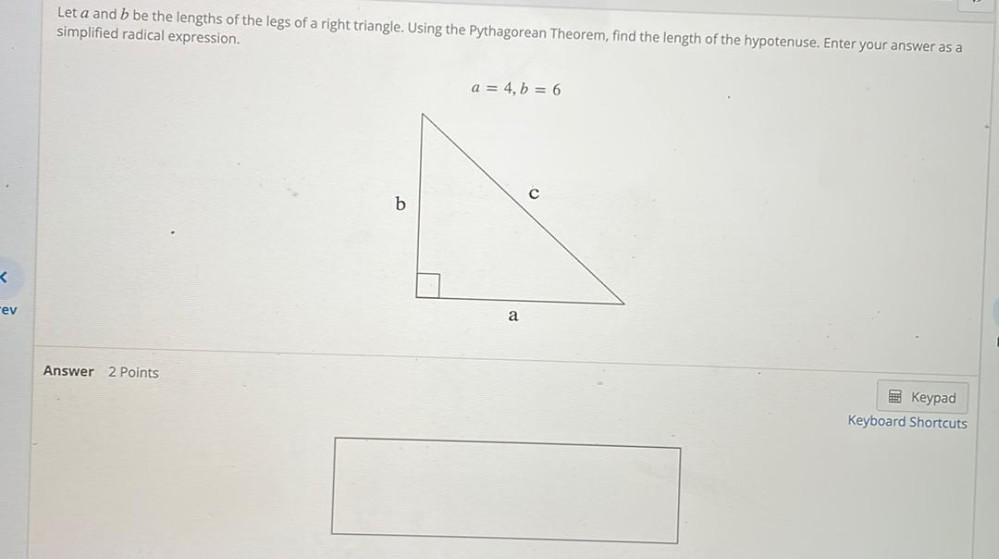Question:

# Let a and b be the lengths of the legs of a right triangle. Using the Pythagorean Theorem, find the length of the hypotenuse. Enter your answer as a simplified radical expression. a = 4, b = 6Let a and b be the lengths of the legs of a right triangle. Using the Pythagorean Theorem, find the length of the hypotenuse. Enter your answer as a simplified radical expression. a = 4, b = 6